# KSEEB Solutions for Class 6 Maths Chapter 1 Knowing Our Numbers Ex 1.3

Students can Download Chapter 1 Algebra Ex 1.3 Questions and Answers, Notes Pdf, KSEEB Solutions for Class 6 Maths helps you to revise the complete Karnataka State Board Syllabus and score more marks in your examinations.

## Karnataka State Syllabus Class 6 Maths Chapter 1 Algebra Ex 1.3

Question 1.
Estimate each of the following using general rule:
a. 730 + 998
b. 796 – 314
c. 12,904 + 2,888
d. 28,292 – 21,496
Make ten more such examples of addition, subtraction and estimation of their outcome.
a. 730 + 998 :-
By rounding off to hundreds, 730rounds off to 7.00 and 998 rounds off to 1000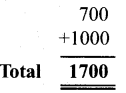The addition of these two number is 1700.b. 796 – 314
By rounding off to hundreds, 796 rounds off to 800 and 314 rounds off to 300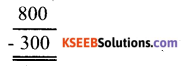Total 500
The difference of these two number is 500.

c. 12,904 + 2,888:-
By rounding off to thousands, 12904 rounds off to 13000 and 2822 round off to 3000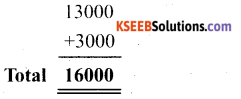The addition of these two number is 16,000.

d. 28,292 – 21,496:-
By rounding off to nearest thousands, 28296 rounds off to 28000 and 21496 rounds off to 21000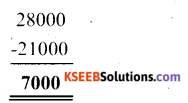The difference of these two numbers is 7000.Question 2.
Give a rough estimate (by rounding off to nearest hundreds) and also a close estimate (by rounding off to nearest tens):
a. 439 + 334 + 4317
b. 1,08,734 – 47,599
c. 8325 – 491
d. 4,89,348 – 48,365
Solution:
a. 439 + 334+ 4317:-
Rounding off to nearest hundreds, 439, 334 and 4317 may be sounded may be rounded off to 400, 300 and 4300 respectively.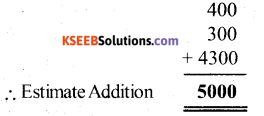Rounding off to nearest tens, 439, 334 and 4317 may be rounded off to 440, 330 and 4320 respectively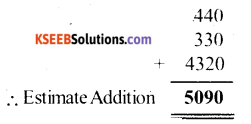b. 1,08,734 – 47,599
Rounding off to hundreds, 108,734 and 47,599 may be rounded off to 1,08,700 and 47.600 respectively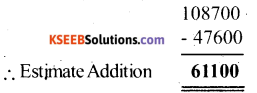Rounding off to tens 1,08,734 and 47,599 may be rounded off to 1,08,730 and 47,600 respectively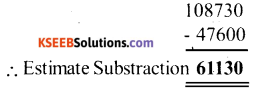c. 8325 – 491:-
Rounding off to hundreds 8325 and 491 may be rounded off to 8330 and 490 respectively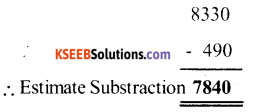d. 4,89,348-48,365:-
Rounding off to hundreds, 489348 and 48365 may be rounded off to 489300 and 48400 respectively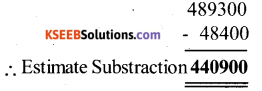Rounding off to tens 489348 and 48365 may be rounded off to 489350 and 48370 respectively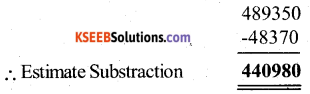Question 3.
Estimate the following products using general rule:
a. 578 × 161
b. 5281 × 3491
c. 1291 × 592
d. 9250 × 29 make four more such examples.
Solution:
For the following roundoff nearly Thousand & Hundreds
a. 578 × 161
Rounding off by general rule, 598 and 161 may be rounded off to 600 and 200 respectively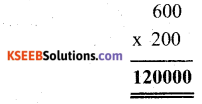OR
600 × 200 = 1,20,000
∴ Product Estimation = 1,20,000

b. 281 × 3491
Rounding off by general rule, 5281 and 3491 may be rounded off to 5000 and 3000 respectively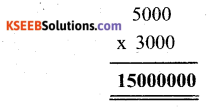5000 × 3000 = 15000000
OR
∴ Product Estimation = 15000000c. 1291 × 592
Rounding off by general rule, 1291 and 592 may be rounded off to 1000 and 600 respectively.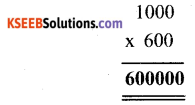OR
1000 × 600 = 600000
∴ Product Estimation = 6,00,000

d. 9250 × 29
Rounding off by general rule, 9250 and 29 may be rounded off to 9000 and 30 respectively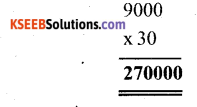OR
9000 × 30 = 270000
∴ Product Estimation = 2,70,000